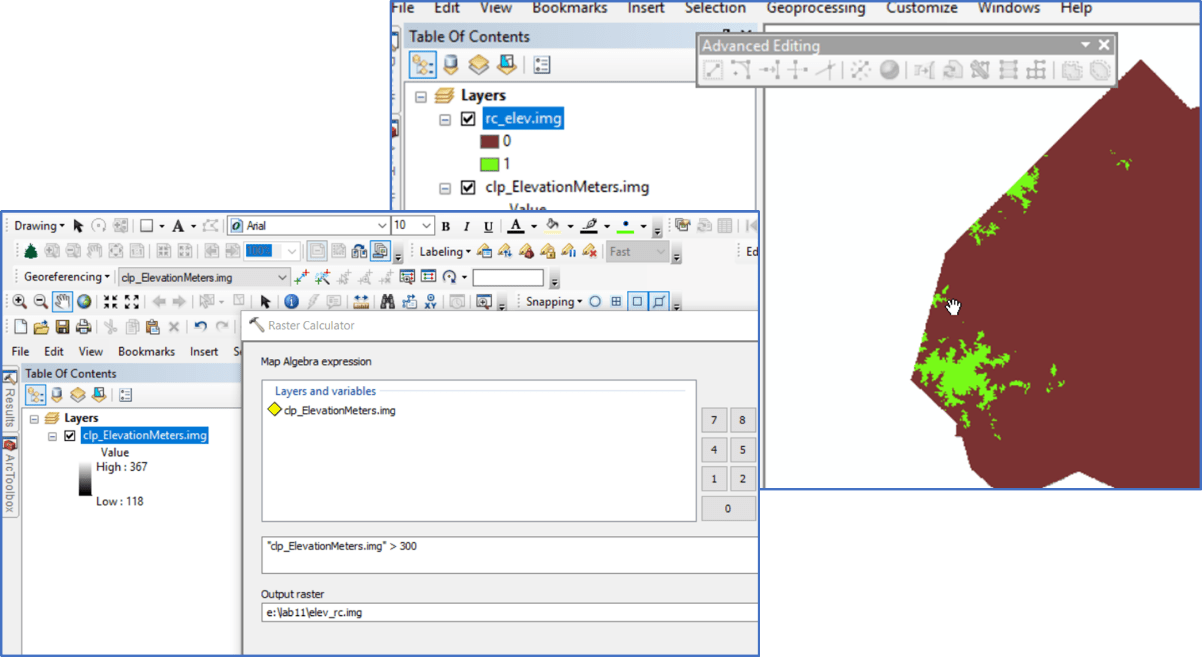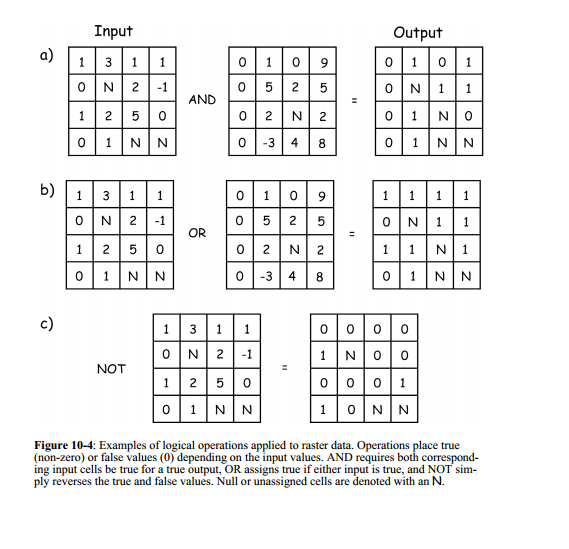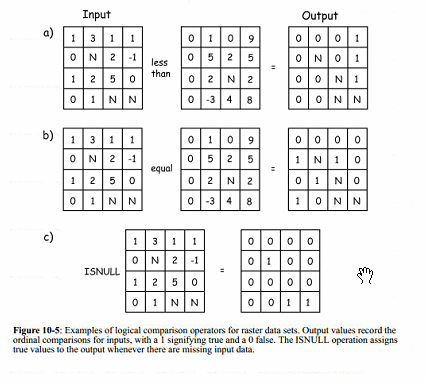# Raster Analysis I

Raster Analysis (Bolstad pages 444 – 474)

Consider the following management need. How would you approach this analysis?LOCAL FUNCTIONS: Processes the raster surface cell-by-cell

Perform Map Algebra with Raster Calculator and/or the Spatial Analyst tools found in the ArcToolbox

You can apply mathematical functions to individual or multiple layers*Syntax might vary among software

Logical operators, >, <, ==, | can be applied in the Raster Calculator. Comparison can among an layer and a constant or among multiple layers. For exampleORORReclassification is used when you want to replace the values in an input raster with new rasters. This could be due to, among others, 1) finding out that the current value has changed since the data was created (land cover type changes from one value to another), or 2) you may want to simplify the information in a raster (reduce urban-low, urban-med, urban-high classes into one urban class), or to 3) assign priority or preference (best to build a road on sites with a low slope, but can build on sites with medium slope), or you 4) may want to remove or add NoData values to control the cells included in the analysis.

Reclassify by individual values (http://desktop.arcgis.com/en/arcmap/latest/tools/spatial-analyst-toolbox/reclass-by-individual-values.htm)

Reclassify by range of values (http://desktop.arcgis.com/en/arcmap/latest/tools/spatial-analyst-toolbox/reclass-by-ranges-of-values.htm)

RECLASSIFY (SPATIAL ANALYST) Tool

CON (SPATIAL ANALYST) Tool

CON (Raster Calculator)

CON(test, new value if true, new value if false)

RASTER OVERLAYS are performed through a series of reclassifications and algebraic functions. Care must be taken, however, that the results must not contain ambiguous results. Consider the following:

Your inputs are two raster layers representing land cover and slope. You want to locate the areas that are Agriculture fields with low slope. You reclassify your landcover so all agriculture cells have a VALUE of 1 and all other cells have a VALUE of 0, and you reclassify your landcover so all low slope cells have a VALUE of 1 and all others have a VALUE of 0. Knowing that the Raster Calculator operates on a cell-by-cell basis,

• What do you know if you add the two reclassified layers together?
• What do you know if you multiply the two reclassified layers together?
• What do you know if you multiply the raster landcover layer by the reclassified slope layer?